Next: Polyhedral Computation Codes Up: Linear Programming Previous: Linear Programming   Contents

## What is LP?

A linear programming (abbreviated by LP) is to find a maximizer or minimizer of a linear function subject to linear inequality constraints. More precisely,

 maximize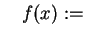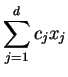(12) subject to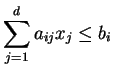for all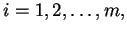(13)

whereis a given rationalmatrix,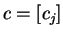and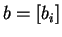are given rational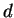- and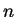-vector. We often write an LP in matrix form:

 maximize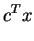(14) subject to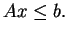(15)

Theoretically every rational LP is solvable in polynomial time by both the ellipsoid method of Khachian (see [Kha79,Sch86]) various interior point methods (see [Kar84,RTV97]). The well-known simplex method of Dantzig (see [Dan63,Chv83]) has no known polynomial variants. In practice, very large LPs can be solved efficiently by both the simplex method and interior-point methods. For example, it is very easy on a standard unix station to solve an LP with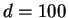and, while the vertex enumeration/convex hull computation of the same size is simply intractable. There are many commercial codes and public codes available. See the LP FAQ [FG]. Two excellent books on LP are Chvatal's textbook [Chv83] and Schrijver's researcher's bible'' [Sch86].Next: Polyhedral Computation Codes Up: Linear Programming Previous: Linear Programming   Contents
Komei Fukuda 2004-08-26